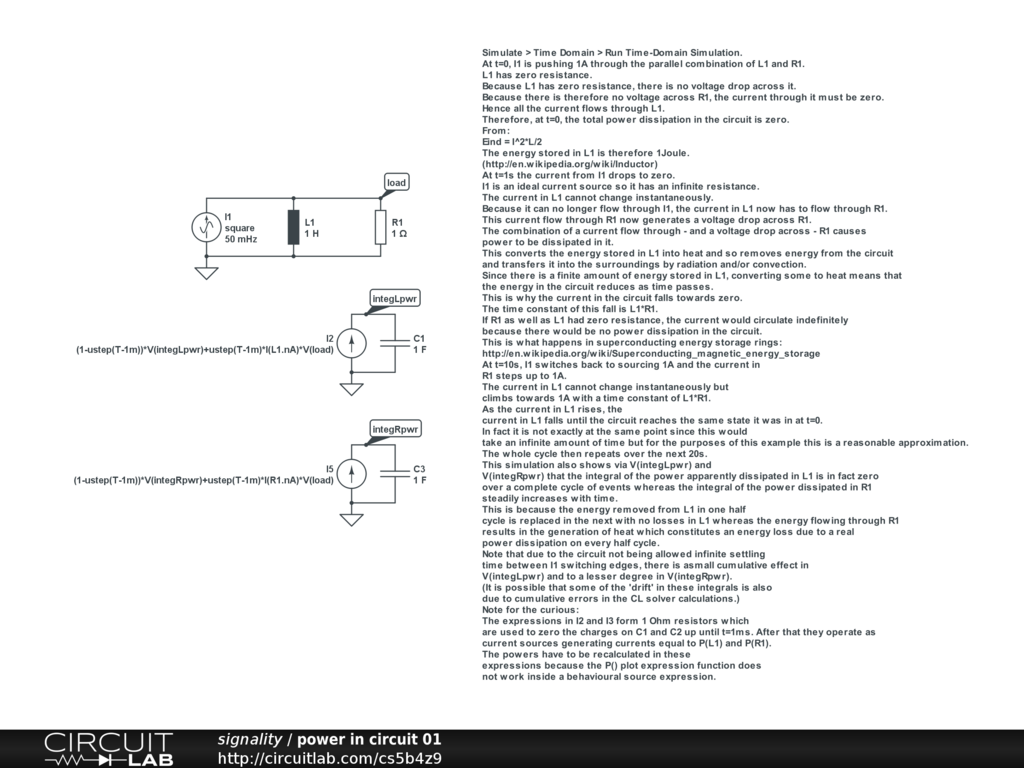## Power Produced by Circuit

 https://www.circuitlab.com/circuit/gyu8ur/power-produced-by-circuit/ Where does the additional power come from? by zenos October 14, 2012 Does this help?by signality October 24, 2012 Thank you for replying to my question. I will have to study your response further. by zenos October 25, 2012 One way of looking at it is that the power dissipated in resistive components as calculated by V*I is always positive (+ve) whereas the calculation of V*I for an inductor (or a capacitor) can be positive or negative (-ve). Energy is the sum of power over time. This is the integral of power which is the same as the area under the power curve. If the product of V*I is always +ve then the area under the curve or the integral increases. If the product of V*I is -ve then the area under the curve is also -ve. If the product of V*I alternates +ve and -ve, as with an inductor or capacitor then over a whole cycle, the +ve area under the V*I curve in one part of the cycle will be cancelled out by the -ve area under the V*I curve in the rest of the cycle. This is because pure inductors and capacitors store energy. Resistors do not. So the V*I power you can get out of an inductor or capacitor can only be equal to the V*I power you put into it. The "extra" power you see in your original sim appears because your sim does not show you what happens when the supply is turned on from zero: this happens before your sim starts. If you use one of the various possible methods to ramp up the supply from zero after your sim starts and then carefully study the area under the V*I curves for components around the circuit and you'll find that the integral of the reactive power through the inductor sums to zero. Actually given the limitations of the solvers in CL, the sum to zero may not be exact but you should get the idea. Also beware that real L's and C's are not ideal. They have various resistive losses that do dissipate real power so in real LC circuits energy does get lost in the circuit as heat. Except in superconductors ... but then you can argue that it takes a lot of energy to keep the superconductors cold enough to store the energy without losses ... :) Some basic background on power in AC circuits: http://en.wikipedia.org/wiki/AC_power by signality October 26, 2012